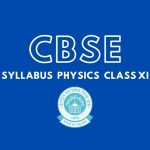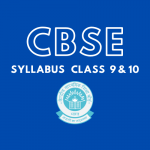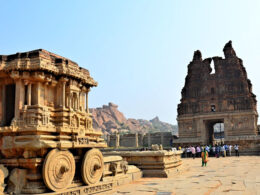# Class XI Physics Practical | New List Of Experiments 2021-21

## Physics Practical List of Experiments for Students Of Class XI

Class XI Physics Practical Experiments are decided by CBSE along wise the CBSE Syllabus. Here is the list of experiments and activities for Class XI Physics Practical Exam.

SECTION—A

### Lists Of Experiments | Physics Class XI

1. To measure diameter of a small spherical/cylindrical body and to measure internal diameter and depth of a given beaker/calorimeter using Vernier Callipers and hence find its volume.
2. To measure diameter of a given wire and thickness of a given sheet using screw gauge.
3. To determine volume of an irregular lamina using screw gauge.
4. To determine radius of curvature of a given spherical surface by a spherometer.
5. To determine the mass of two different objects using a beam balance.
6. To find the weight of a given body using parallelogram law of vectors.
7. Using a simple pendulum, plot its L-T2 graph and use it to find the effective length of second’s pendulum. To study variation of time period of a simple pendulum of a given length by taking bobs of same size but different masses and interpret the result.
8. To study the relationship between force of limiting friction and normal reaction, and to find the co- efficient of friction between a block and a horizontal surface.
9. To find the downward force, along an inclined plane, acting on a roller due to gravitational pull of the earth and study its relationship with the angle of inclination 0 by plotting the graph between force and sin0.

List of Activities | Physics Class XI

1. To make a paper scale of given the least count, e.g., 0.2 cm, 0.5 cm.
2. To determine mass of a given body using a metre scale by principle of moments.
3. To plot a graph for a given set of data, with proper choice of scales and error bars.
4. To measure the force of limiting friction for rolling of a roller on a horizontal plane.
5. To study the variation in range of a projectile with angle of projection.
6. To study the conservation of energy of a ball rolling down on an inclined plane (using a double inclined plane).
7. To study dissipation of energy of a simple pendulum by plotting a graph between square of amplitude and time.

SECTION—B

### List of Experiments | Physics Class XI

1. To determine Young’s modulus of elasticity of the material of a given wire.
2. To find the force constant of a helical spring by plotting a graph between load and extension.
3. To study the variation in volume with pressure for a sample of air at constant temperature by plotting graphs between P and V, and between P and 1/V.
4. To determine the surface tension of water by capillary rise method.
5. To determine the coefficient of viscosity of a given viscous liquid by measuring terminal velocity of a given spherical body.
6. To study the relationship between the temperature of a hot body and time by plotting a cooling curve.
7. To determine specific heat capacity of a given solid by method of mixtures.
8. To study the relation between frequency and length of a given wire under constant tension using sonometer.
9. To study the relation between the length of a given wire and tension for constant frequency using sonometer.
10. To find the speed of sound in air at room temperature using a resonance tube by two resonance positions.

List of Activities | Physics Class XI

1. To observe change of state and plot a cooling curve for molten wax.
2. To observe and explain the effect of heating on a bi-metallic strip.
3. To note the change in level of liquid in a container on heating and interpret the observations.
4. To study the effect of detergent on surface tension of water by observing capillary rise.
5. To study the factors affecting the rate of loss of heat of a liquid.
6. To study the effect of load on depression of a suitably clamped metre scale loaded at (i) its end (ii) in the middle.
7. To observe the decrease in pressure with increase in velocity of a fluid.

## Physics Practical List of Experiments for Visually Impaired Students Of Class XI

Note: Same Evaluation scheme and general guidelines for visually impaired students as given for Class XII may be followed.

### A. Items for Identification/Familiarity of the apparatus for assessment in practicals (All experiments)

• Spherical ball, Cylindrical objects, vernier calipers, beaker, calorimeter, Screw gauge, wire, Beam balance, spring balance, weight box, gram and milligram weights, forceps, Parallelogram law of vectors apparatus, pulleys and pans used in the same ‘weights’ used, Bob and string used in a simple pendulum, meter scale, split cork, suspension arrangement, stop clock/stop watch, Helical spring, suspension arrangement used, weights, arrangement used for measuring extension, Sonometer, Wedges, pan and pulley used in it, ‘weights’ Tuning Fork, Meter scale, Beam balance, Weight box, gram and milligram weights, forceps, Resonance Tube, Tuning Fork, Meter scale, Flask/Beaker used for adding water.

### B. List of Practicals | Physics Class XI

1. To measure diameter of a small spherical/cylindrical body using vernier calipers.
2. To measure the internal diameter and depth of a given beaker/calorimeter using vernier calipers and hence find its volume.
3. To measure diameter of given wire using screw gauge.
4. To measure thickness of a given sheet using screw gauge.
5. To determine the mass of a given object using a beam balance.
6. To find the weight of a given body using the parallelogram law of vectors.
7. Using a simple pendulum plot L-T and L-T2 graphs. Hence, find the effective length of second’s pendulum using appropriate length values.
8. To find the force constant of given helical spring by plotting a graph between load and extension.
9. (i) To study the relation between frequency and length of a given wire under constant tension using a sonometer. (ii) To study the relation between the length of a given wire and tension, for constant frequency, using a sonometer.
10. To find the speed of sound in air, at room temperature, using a resonance tube, by observing the two resonance positions.

Note: The above practicals may be carried out in an experiential manner rather than recording observations.

Previous Article## New CBSE Physics Syllabus - Class XI | Theory & Practical

Next Article## New CBSE Secondary Curriculum Class IX-X | CBSE Syllabus Class 9 & 10 PDFs

##### Related Posts## Pioneering Archaeologists of India: Uncovering Ancient Mysteries

Picture this: a land steeped in history, where centuries-old secrets lie beneath the earth’s surface, waiting for the…## Aerial Metagenomics: Revealing the Microscopic Marvels Above Us

Table of Contents Show The Marvels of Aerial MetagenomicsThe Technology Behind Aerial MetagenomicsChallenges and Future HorizonsIn Conclusion Aerial…